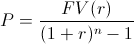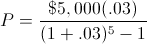# Annuity Payment - FVThe annuity payment formula shown above is used to calculate the cash flows of an annuity when future value is known. An annuity is denoted as a series of periodic payments.

The annuity payment formula shown here is specifically used when the future value is known, as opposed to the annuity payment formula used when present value is known. There are not only mathematical differences between calculating an annuity when present value is known and when future value is known, but also differences in the real life application of the formulas.

For example, if an individual is wanting to calculate the payments on a loan, the original loan balance would be considered the present value and the payment formula would accommodate this known variable. However, if an individual is wanting to calculate how much they need to save per year in an interest bearing account to have a certain balance after a specific period of time, then this wanted balance would be considered the future value. The latter example would use the annuity payment using future value formula as the balance is increasing instead of decreasing.

## Example of Annuity Payment Using Future Value Formula

An example of the annuity payment formula using future value would be an individual who would like to calculate the amount they would need to save per year to have a balance of \$5,000 after 5 years. For this example, it is assumed that the effective rate per year would be 3%.

It is important to remember that the rate per period and the occurrence of periodic payments need to match. For example, if the payments are made monthly, then the rate used would be the effective monthly rate.

Using the variables from this example, the equation for annuity payments would beAfter solving, the amount needed to save per year is \$941.77. Real amounts may vary by cents due to rounding.

It is worth noting that the formula for this example is an ordinary annuity. This means that the first cash flow or payment is not received until after the first period. In this example, the end of the first period is one year away. If a payment or cash flow is saved immediately, then the annuity due for future value formula would be used.

## How is the Annuity Payment Formula Derived from Future Value?

The annuity payment formula using future value can be found by first looking at the future value of an annuity formula:This formula shown directly above can be rearranged to solve for the payment. After rearranging, the formula above will show:This formula can be further simplified by multiplying the numerator times the reciprocal of the denominator, as shown in the formula at the top of the page.

*Disclaimer:This site is compensated for sales made through affiliate links. There is no additional cost to you and it keeps the site running.

Resources

Financial Education ←

Increase your knowledge in Finance and other topics through courses at Udemy. Financial course topics include Personal Finance, Financial Analysis, Investing, Tech Analysis, and more.

Start Learning Today! Udemy courses up to 50% off (affiliate)

Banking/Investment ←

Invest your spare change (microinvesting), save for retirement, and get bonuses while you shop through Acorns

Start Now! Get a \$5 Sign Up Bonus for Acorns today! (Tier) (affiliate)

New to Finance?